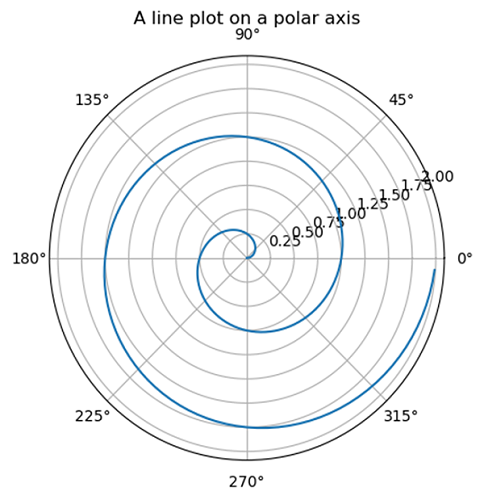# Python | Polar Plot in Python using Matplotlib

In this tutorial, we are going to learn how to create a Polar Plot in python using matplotlib?
Submitted by Anuj Singh, on August 03, 2020

There is a syntax defined for Polar Plot in matplotlib.pyplot as shown below:

```matplotlib.pyplot.subplot(111, projection='polar')
matplotlib.pyplot.plot(theta, r)
```

This is an example showing the implementation of polar plot.

Example:## Python code for polar plot in python using matplotlib

```import numpy as np
import matplotlib.pyplot as plt

r = np.arange(0, 2, 0.01)
theta = 2 * np.pi * r

plt.subplot(111, projection='polar')
plt.plot(theta, r)
plt.title("A line plot on a polar axis", va='bottom')
plt.show()
```

Output:

```Output is as figure
```

Languages: » C » C++ » C++ STL » Java » Data Structure » C#.Net » Android » Kotlin » SQL
Web Technologies: » PHP » Python » JavaScript » CSS » Ajax » Node.js » Web programming/HTML
Solved programs: » C » C++ » DS » Java » C#
Aptitude que. & ans.: » C » C++ » Java » DBMS
Interview que. & ans.: » C » Embedded C » Java » SEO » HR
CS Subjects: » CS Basics » O.S. » Networks » DBMS » Embedded Systems » Cloud Computing
» Machine learning » CS Organizations » Linux » DOS
More: » Articles » Puzzles » News/Updates